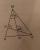Mast

Mast has 17 m long shadow on a slope rising from the mast foot in the direction of the shadow angle at angle 9.3°. Determine the height of the mast, if the sun above the horizon is at angle 44°30'.

Result

h =  19.23 m

Solution:Leave us a comment of example and its solution (i.e. if it is still somewhat unclear...):Be the first to comment!To solve this example are needed these knowledge from mathematics:

Do you want to convert length units? See also our right triangle calculator. Most natural application of trigonometry and trigonometric functions is a calculation of the triangles. Common and less common calculations of different types of triangles offers our triangle calculator. Word trigonometry comes from Greek and literally means triangle calculation.

Next similar examples:

1. Cable car 2Cable car rises at an angle 41° and connects the upper and lower station with an altitude difference of 1175 m. How long is the track of cable car?
2. 30-60-90The longer leg of a 30°-60°-90° triangle measures 5. What is the length of the shorter leg?
3. FlowerbedFlowerbed has the shape of an isosceles obtuse triangle. Arm has a size 5.5 meters and an angle opposite to the base size is 94°. What is the distance from the base to opposite vertex?
4. SteepleSteeple seen from the road at an angle of 75°. When we zoom out to 25 meters, it is seen at an angle of 20°. What is high?
5. TreeHow tall is the tree that observed in the visual angle of 52°? If I stand 5 m from the tree and eyes are two meters above the ground.
6. Theorem proveWe want to prove the sentence: If the natural number n is divisible by six, then n is divisible by three. From what assumption we started?
7. LiftThe largest angle at which the lift rises is 16°31'. Give climb angle in permille.
8. CosineThe point (8, 6) is on the terminal side of angle θ. cos θ = ?
9. Reference angleFind the reference angle of each angle:
10. BuildingThe building I focused at an angle 30°. When I moved 5 m building I focused at an angle 45°. What is the height of the building?
11. ReflectorCircular reflector throws light cone with a vertex angle 49° and is on 33 m height tower. The axis of the light beam has with the axis of the tower angle 30°. What is the maximum length of the illuminated horizontal plane?
12. Right triangleCalculate the length of the remaining two sides and the angles in the rectangular triangle ABC if a = 10 cm, angle alpha = 18°40'.
13. High wallI have a wall 2m high. I need a 15 degree angle (upward) to second wall 4 meters away. How high must the second wall?
14. CosineCalculate the cosine of the smallest internal angle in a right-angled triangle with cathetus 3 and 8 and with the hypotenuse 8.544.
15. CotangentIf the angle α is acute, and cotg α = 1/3. Determine the value of sin α, cos α, tg α.
16. Obtuse angleThe line OH is the height of the triangle DOM, line MN is the bisector of angle DMO. obtuse angle between the lines MN and OH is four times larger than the angle DMN. What size is the angle DMO? (see attached image)
17. TrigonometryIs true equality? ?